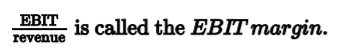# Application of Dupont analysis of return on equity

Dupont system of analysis (1)

• Used to analyze return on equity (2) (ROE)
• Analyst can see the impact of
• Leverage
• Profit margins
• Turnover on shareholder returns
• Two variants
• Original three-part approach (3)
• Break down a very important ratio into 3 key components
• Most important equation in ratio analysis
• If ROE is low, the reason should be
• Poor profit margin (weak operation)
• Poor asset turnover (weak investment)
• Too little leverage (weak financing)
• Extended five-part system  (4)

Original approach

• ROE:
••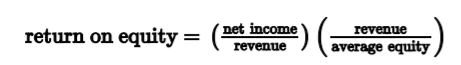•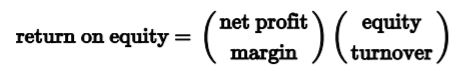•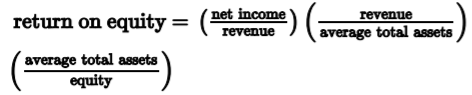•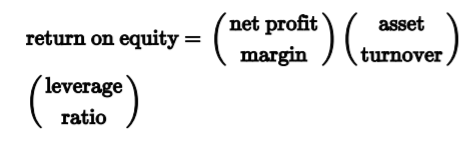Extended Dupont equation (4)

• Take net profit margin and breaks down further
•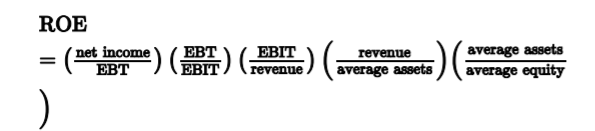• Tax burden
•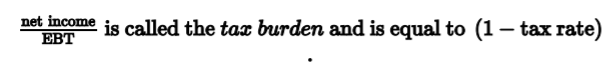• Interest burden
•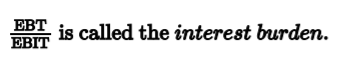• Leverage rise when interest burden rise
• EBIT margin
•